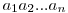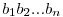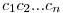# Hex, Bugs and More Physics | Emre S. Tasci

a blog about physics, computation, computational physics and materials…

## A Mathematica Example for Information Gain

### November 19, 2007 Posted by Emre S. Tasci

Mathematica Code for Andrew Moore – Information Gain Example This is what I’ve written implementing the two libraries. It calculates the information gain for Andrew Moore’s example in his Information Gain example (which can be accessed from his resourceful website on datamining: http://www.autonlab.org/tutorials/)

You will be needing the two libraries and you can download them from the related entries for Matrix and DataMining procedures.

## DataMining Functions Library for Mathematica

### Posted by Emre S. Tasci

Mathematica Library for DataMining
Functions Library Intended To Be Used InÂ DataMining Procedures
11/2007
Emre S. Tasci
S&C / MSE / 3mE / TUDelftFunctions List (EF: Required External Functions)

• Int DMBooleEST[statement_]
Converts True/False => 1/0
EF – NONE
• Bool DMBooleEST[statement_]
Converts 1/0 => True/False
EF – NONE
• Int DMCondEntropyEST[MatrixIN_,var2_,var1_, posVal1_, posVal2_,probVar1_]
Calculates the Conditional Entropy H(var2|var1)
EF – MSelectEST, DMProbeEST, DMEntropyEST
• Int DMEntropyEST[problist_]
Calculates the entropy given the probability list “problist”
EF – NONE
• Matrix DMGenMatrixEST[dim_,file_]
Generates a Matrix of [dim,dim] dimension and writes this matrix to the “file” file. Returns the matrix generated.
EF – DMBooleEST, DMUnitizeEST
• List DMInfGainEST[MatrixIN_,varno_]
Calculates the Information Gain related to the “varno” variable wrt to the remaining variables.
EF -Â MDiffElementEST , MSwapEST, DMCondEntropyEST, DMEntropyEST, DMProbeEST
• Vector DMProbeEST[MatrixIN_,varno_,varlist_]
Calculates the probabilities of the variables’ possible outcomes.
EF – NONE;

Important!!! ————————————

Assumed form of the MatrixIN: ;(i.e., values for the same variable are stored in ROWS, NOTÂ in COLUMNS!)

## Matrix Functions Library for Mathematica

### Posted by Emre S. Tasci

Mathematica Library for additional Matrix Functions

Functions Library Intended To Be Used In Matrix Procedures 11/2007 Emre S. Tasci S&C / MSE / 3mE / TUDelft Functions List (EF: Required External Functions)

• List MDiffElementEST[matrixIN_,varno_]
Returns the list of the different elements in the (varno). row of the specified matrixIN
EF – NONE
• List MPolluteEST[mx_,row_,ratio_]
Assigns a 0 or 1 (with equal probabilities) to the randomly selected "ratio" of the elements of the matrix "mx" and returns the new "polluted" matrix.If "mx" is a List, then "row" is not relevant.
*EF – NONE;
• List MSelectEST[MatrixIN_,varno1_,varval1_, varno2_]
Returns the list of "varno2" variables from the "MatrixIN" where "varno1" values equal to "varval1"
EF – NONE / AppendRows from LinearAlgebra`MatrixManipulation` (Optional)
• List MSwapEST[MatrixIN_,row1_,row2_]
Swaps the rows "row1" and "row2" in matrix "MatrixIN"
EF – NONE

Important!!!

Assumed form of the MatrixIN: ;(i.e., values for the same variable are stored in ROWS, NOT in COLUMNS!)